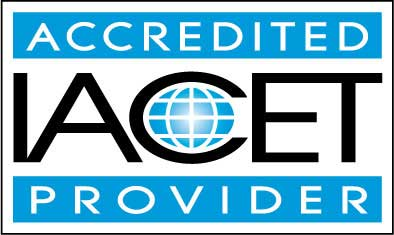Course SyllabusCourse Description

At a minimum, a basic understanding of chemistry is needed for it offers a foundation for comprehending the inner workings of biology (how cells function and the behavior of organisms), as well as, the ecological relationships that exist between organisms and their environment.

In short, chemistry offers us a way of understanding the world in which we live.

Chemistry provides us with insights into how our bodies function; the ingredients that are contained within the foods we eat; the reason cars, planes and trains are able to run as efficiently as they do; the manner in which computers are built and operate; and the materials used to construct our homes and buildings within our communities.

Basically, chemistry is a part of almost everything we do for everything is comprised of chemical compounds. The claim that chemistry is everywhere is, thus, entirely accurate.

Chemistry encompasses a multitude of specialized sub-disciplines which have proven highly useful to chemistry as they have provided for the following: the production and testing of stronger materials, creation of pharmaceuticals to treat disease, and the study of life processes.
• Completely Online
• Self-Paced
• 6 Months to Complete
• Start Anytime
• PC & Mac Compatible
• Android & iOS Friendly
• Accredited CEUsLearning Outcomes

By successfully completing this course, students will be able to:
• Define atoms, molecules, and ions.
• Describe chemical foundations and stoichiometry.
• Identify gases and thermochemistry.
• Describe atomic structure and periodicity.
• Describe bonding and the Valence Bond Theory.
• Summarize properties and solutions.
• Describe chemical equilibrium.
• Summarize spontaneity, entropy, and free energy.
• Summarize the nucleus, a chemist's view.
• Summarize transition metals and coordination chemistry.
• Summarize organic chemistry.
• Demonstrate mastery of lesson content at levels of 70% or higher.

Assessment Guide

Assessment Points
Lesson 1 Assignment 25 points
An Introduction 5 points
Lesson 1 Exam 10 points
Lesson 2 Assignment 25 points
Lesson 2 Exam 10 points
Lesson 3 Assignment 25 points
Lesson 3 Exam 10 points
Lesson 4 Assignment 25 points
Lesson 4 Exam 10 points
Lesson 5 Assignment 25 points
Lesson 5 Exam 10 points
Lesson 6 Assignment 25 points
Lesson 6 Exam 10 points
Lesson 7 Assignment 25 points
Lesson 7 Exam 10 points
Lesson 8 Assignment 25 points
Lesson 8 Exam 9 points
Lesson 9 Assignment 25 points
Lesson 9 Exam 10 points
Lesson 10 Assignment 25 points
Lesson 10 Exam 10 points
Lesson 11 Assignment 25 points
Lesson 11 Exam 10 points
Lesson 12 Assignment 25 points
Lesson 12 Exam 10 points
Lesson 13 Assignment 25 points
Lesson 13 Exam 10 points
Lesson 14 Assignment 25 points
Lesson 14 Exam 10 points
Lesson 15 Assignment 25 points
Lesson 15 Exam 10 points
Lesson 16 Assignment 25 points
Lesson 16 Exam 10 points
The Final Exam 46 points